Next: 1.3.2 Resistance Increase and Up: 1.3 Empirical and Semi-Empirical Previous: 1.3 Empirical and Semi-Empirical

## 1.3.1 Black's Equation

Based on a very simple model, Black [45,46,47] was the first to derive an expression for the time to failure of a metal line subjected to electromigration. He considered that the mean time to failure,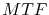, is inversely proportional to the rate of mass transport,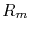,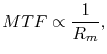(1.1)

and that the rate of mass transport is proportional to the momentum transfer between thermally activated ions and conducting electrons,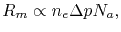(1.2)

where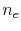is the density of conducting electrons,is the momentum transfer from the electrons to the metal atoms, and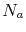is the density of thermally activated ions. Furthermore, assuming that both the electron density as well as the momentum transfer are proportional to the current density,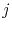,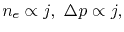(1.3)

and that the activated ions follow an Arrhenius law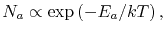(1.4)

the mean time to failure is modeled as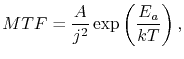(1.5)

where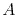is a constant which comprises the material properties and the geometry of the interconnect [45,47],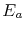is the activation energy,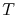is the temperature, and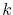is Boltzmann's constant. It was observed that not all experimental results followed (1.5), but they could be fitted by allowing a variable current density exponent,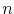, which can be experimentally determined. Therefore, Black's equation was modified to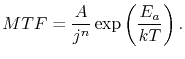(1.6)

It is interesting to note that the original equation (1.5) predicted a failure time proportional to the inverse square of the current density, even though mass transport due to electromigration had been shown to be linearly dependent on the current density . This issue was discussed by several authors [48,49], however, the explanation for the square dependence was elucidated only several years later, when various theoretical works [50,51,52,53,54,55] considerably contributed to a better understanding of the electromigration behavior. An exponent close to 1 indicates that the lifetime is dominated by the void growth mechanisms, i.e. the time for a void to grow and lead to failure represents the major portion of the lifetime [51,52], while a value close to 2 indicates that void nucleation is the dominant phase of the electromigration lifetime [50,53,54,55].

Since equation (1.6) connects the EM time to failure with the material transport mechanism via the activation energy parameter, and the current density dependence via the exponent. Thus, these parameters can be experimentally determined from the accelerated tests. Taking the logarithm of (1.6) yields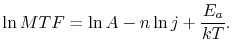(1.7)

Testing an interconnect at several temperatures, the mean time to failure can be plotted as a function of the temperature, as shown in Figure 1.4(a). For a constant current density, (1.7) shows that the logarithm of the mean time to failure varies linearly with the inverse of temperature, therefore, the activation energy can be extracted from the measurement of the angular coefficient in Figure 1.4(a). The current density exponentcan be determined in a similar way. Varying the current density and keeping the test temperature constant, the coefficient angular of the plot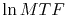versus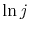determines the current density exponent, as shown in Figure 1.4(b).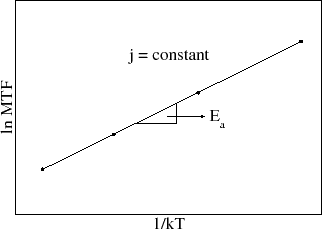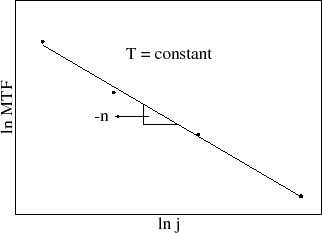(a)                                                                              (b)

This method of parameter extraction together with equation (1.6) has been used for lifetime estimation and extrapolation to operating conditions for 40 years now. However, in a recent publication Lloyd  discussed this application of the modified equation (1.6) and concluded that it may lead to significant errors in the lifetime extrapolation. These errors arise from the assumption that the fitting parameters,, andobtained under accelerated tests are also valid at real operating conditions, so that they can be directly applied for the lifetime extrapolation. As Lloyd  shows, the experimental determination of the above parameters does not consider important additional temperature and also pre-existing stress dependences, which yields incorrect parameter values and, consequently, lifetime extrapolation.

Black's equation provides useful insight into electromigration failure, however, it does not allow a thorough understanding of the underlying physics related to the electromigration behavior for which more sophisticated physically based models are required.Next: 1.3.2 Resistance Increase and Up: 1.3 Empirical and Semi-Empirical Previous: 1.3 Empirical and Semi-Empirical

R. L. de Orio: Electromigration Modeling and Simulation